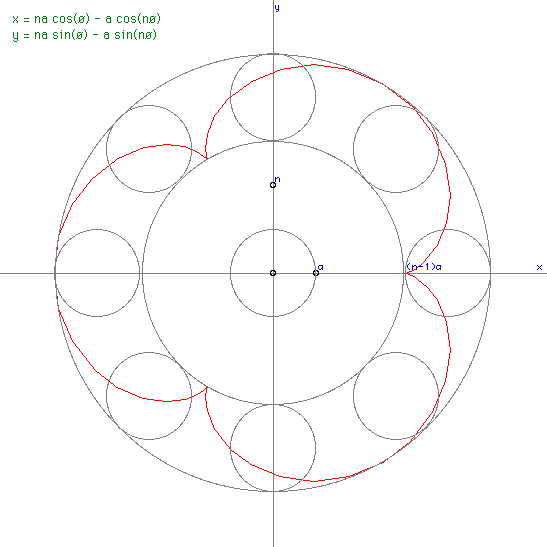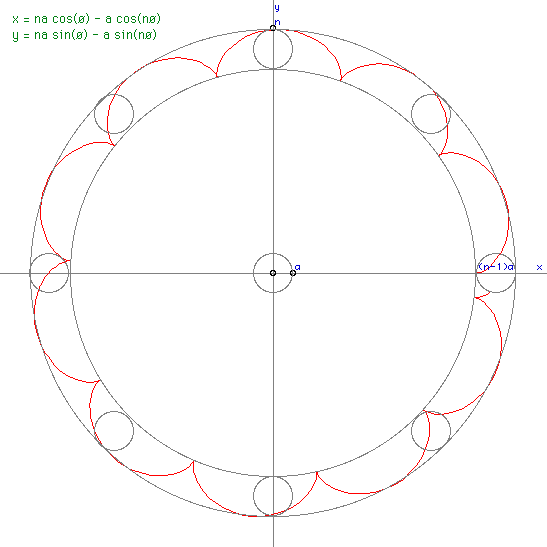# Epicycloid

Formed as the locus of a point on a circle (radius r1) rotated (without slipping) on the outside of a fixed circle (radius r2) then

x = (r2 + r1) cos(theta) - r1 cos((r2 + r1) theta / r1)
y = (r2 + r1) sin(theta) - r1 sin((r2 + r1) theta / r1)